## Traditional Average & Variance Computation

Suppose you have the following data: 4, 6, 12, 9 and you want to calculate the simple statistics of average (arithmetic mean) and variance, how do you calculate that?

Average =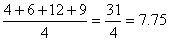Variance =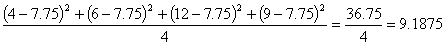Our focus in particular tutorial is for the time average and time variance of the measurement data. Let us use symbols to ease the algebraic manipulation. Symbol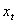denotes the data number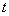-th. The subscript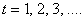represent the order of the data when it comes out. In our example above,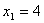,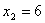,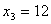and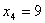. Notation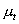represents the average while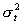represents variance of the-th data. In symbolic notation, we say that the usual way to compute the time average is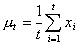(1)

While the time variance is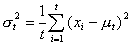(2)

Now let us consider a case when you have a device to measure something, say number of pedestrians passing through a gate of a subway stations. Every measurement time, for example, every minutes, you get the total number of people who pass through that gate. At each time (i.e. every minutes), you get an additional data and you need to calculate those simple statistics of average and variance of the measurement. How do you calculate them?

Let us use the same data as above for our example. The first measurement, give 4 person passed through the gate. The average is the same as the measurement and there is no variance because we have only single data. The second, third and fourth measurement, give 6, 12 and 9 pedestrians respectively who passed through the gate. The average and variance calculation is shown in the table below.

 Time () Measurement () Average () Variance () 1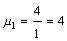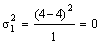2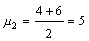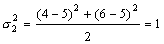3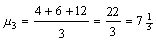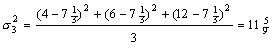4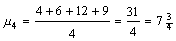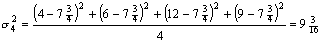So far there is nothing interesting to be discussed about unless if we got so much measurement data. All of sudden, the computation of simple statistics of time average and time-variance become problems .

Experiment with your own input data using the interactive Mean and Variance below. The mean and variance are computed in a traditional way using above formulation. All the data are inputted before the program compute the mean and variance.
To use the program: Type your input data in the box below, separated by comma or space. Only numbers input are accepted. Then click "Compute Mean and Variance" button. Alternatively, click Random Input button repeatedly to create simulated data. To restart with the example data, click "Reset" button. The chart may not work fully if you use Internet Explorer; It is recommended you use FireFox or Google Chrome for better browsing experience.

Preferable reference for this tutorial is

Teknomo, Kardi. (2006) Recursive Average and Variance.
http://people.revoledu.com/kardi/tutorial/RecursiveStatistic/index.html